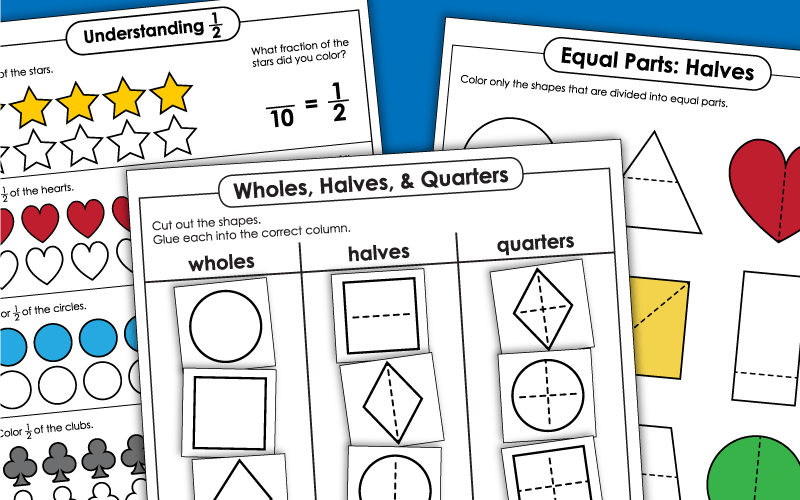# Fractions: Halves & Quarters Only (Very Basic)

These printable activities can be used for teaching fractions with halves and quarters only.

These are the most basic level of fraction worksheets. If you're looking for fractions with numerators and denominators between 1 and 10, please jump over to our main fraction worksheets page.## Halves

Cut out the shapes. Glue the ones that are divided into halves into the halves column of the t-chart. Glue the others into the column labeled not halves.
Part 1: Color the shapes that are divided evenly in half. Part 2: Shade one-half of each shape. Part 3: Draw lines to divide the four shapes in half.
Color or shade half of each set of shapes. Tell how many you colored and write the amount as a fraction.
Color on the shapes that are divided into equal parts on this printable worksheet.

## Quarters (Fourths)

Part 1: Color the shapes that are divided into quarters. Part 2: Shade one-fourth of each picture. Part 3: Draw straight lines to divide the shape into four equal parts.

## Halves & Quarters (Mixed)

Cut out the shapes, categorize them on the worksheet, and glue them into the correct columns.
Cut out each shape. Determine whether each one is partitioned into halves or fourths. Glue the shapes in the correct areas of the t-chart.

## Other Basic Fractions

Color the shapes that are divided into thirds and draw an "X" over the shapes that are not divided into thirds.
More Fraction Worksheets

My Account
Site Information